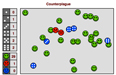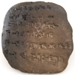### Online multi-media resources

Packs contain a range of resources - short video clips, classroom activities and games, topical issues for discussion, student worksheets, and teacher notes.

*The age ranges suggested are only intended to be a guide to the level of the resources.  Some may well be suitable for younger or older students.

 Topics covered Age ranges* Curriculum areas Maths and Our Health11-13+ 12-14 13-15 14-16+ 15-17 Percentages, graphs Maths in context, interpreting graphs Probability, interpreting graphs, mathematical modelling Multi-step problems, graphs and equations and formulae, probability Conditional probability, independence and dependence, contingency tables and tree diagrams Disease Dynamics Schools PackIntroduction to the Disease Dynamics Schools' Project Presentations on the maths of epidemic spread Activities, simulations and resources for maths classes 13-16+ Mathematical modelling Interpreting graphs Probability Data collection and interpretation Babylonian MathsThe Maths Archaeologist A Babylonian house Making Babylonian numbers on clay tablets Understanding Babylonian numbers and arithmetic Babylonian triangles 10-12 Number and place value Multiplication, division, inverses Shape and symmetry